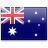S Q T L

S Q T L

S Q T L

S Q T L

S Q T L

S Q L T

S Q L T

L Q S T

S Q T L

S Q L T

S Q L T

Q S L T

L Q S T

Q T S L

Q L S T

S Q T L

L Q T S

Q T L S

T Q L S

T Q S

Q L S T